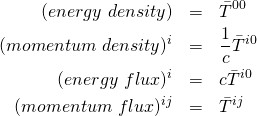Einstein-Maxwell Theory

EQUATIONS OF MOTION

Einstein gravity coupled to the Maxwell electromagnetic field with SI units and MTW sign conventions:

(1)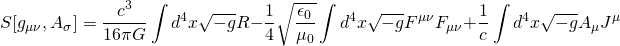where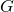is Newton’s constant. The speed of light is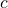, the permittivity of free space is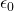, and the permeability of free space is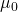; these are related by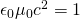. The field strength tensor is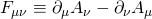and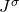is an external charge current.

The variation of the action gives

(2)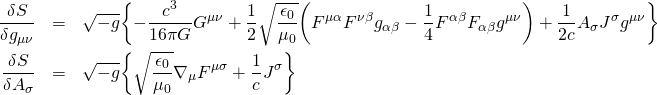so the equations of motion are

(3)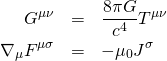with the stress–energy–momentum tensor

(4)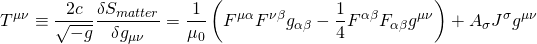Dimensions

Let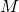,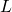,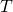, and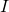denote mass (in kilograms), length (in meters), time (in seconds) and current (in Amperes), respectively. The dimensions of the constants are

(5)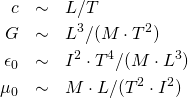Tensor components are coordinate dependent, so their dimensions depend on the dimensions of the coordinates. Let the coordinates have dimensions of length:

(6)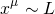Then

(7)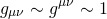and we can raise and lower indices without changing dimensions. Dimensions of other tensors:

(8)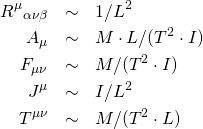ELECTRIC AND MAGNETIC FIELDS

The velocity of an observer is

(9)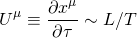where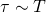is proper time along the worldline. It satisfies the normalization condition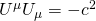. The electric and magnetic fields as seen by this observer are

(10)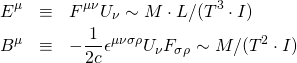The field strength tensor is

(11)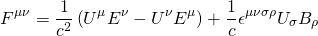The scalar potential and electric charge density are:

(12)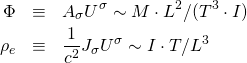The 3-vector potential and the 3-current defined by:

(13)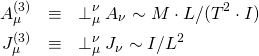where

(14)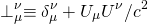is the spatial projection tensor for the observer.

Stress, Energy and Momentum

The stress-energy-momentum tensor is

(15)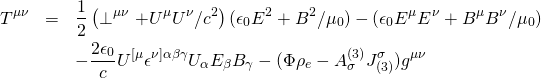The energy density as seen by the observer is

(16)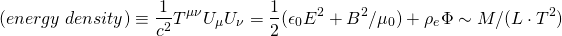The momentum density and energy flux,

(17)are related by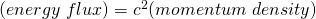. The momentum flux and spatial stress are defined by

(18)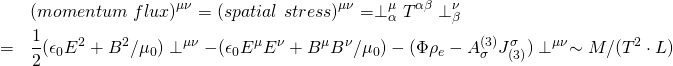Fermi Normal Coordinates

Let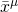denote Fermi normal coordinates (FNC) defined by the observer whose velocity is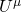. Thus,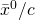is the proper time along the observer’s worldline. The observer defines a triad of vectors,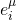that are Fermi-Walker transported along the worldline. These vectors are spatial,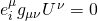, and orthogonal,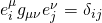. The spatial coordinates of an event,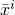, are the coefficients in the expansion of the vector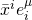that is tangent to the spacelike geodesic that connects the worldline to the event, and has magnitude equal to the length of the geodesic.

In Fermi normal coordinates, the metric on the observer’s wordline is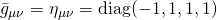and

(19)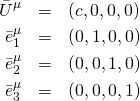so that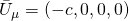. We have

(20)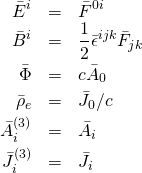where the 3D Levi-Civita tensor is defined by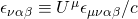. Thus, in FNC,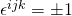if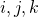is an even/odd permutation of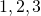. The components of the stress-energy-momentum tensor are:

(21)